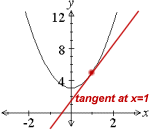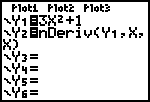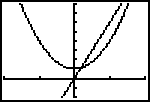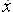Go to contentDrag the red point in the display below to move the tangent line along the quartic (fourth order) curve.

You can see that the gradient function f'(x) of a quartic curve is a cubic curve.

Finally you can investigate higher order curves. In each case, the gradient function f'(x) will be a curve of one order lower.
 Select polynomial order:

## Summary/BackgroundA note about graphic calculators
A graphic calculator may not be allowed in your exam. However you can use one for revision. The TI-83, or similar model, can display graphs and their gradient function for you.
 To set this up, go to the Y= screen. Against Y1= enter a simple curve, 3x^2+1 for example. Against Y2= you should make the following selections: MATH 8 VARS Y-VARS 1 1 then type in ,X,X) The result should be that the Y= screen shows Y1=3x^2+1 and Y2=nDerive(Y1,X,X).Go to the GRAPH screen, to see the graphs of y=3x^2+1 and the gradient function. In this case the gradient function displayed will be that of y=6x. The calculator cannot tell you this though. You will have to work that out for yourself.If now you change the equation against Y1, the calculator will automatically show its derivative.• The function that gives the gradient at any point on a given curve is called the gradient function for that curve
• If the curve is given by y=f(x), then the gradient function is often denoted by f'(x).
• If f(x) is quadratic, then f'(x) is linear
• If f(x) is cubic, then f'(x) is quadratic
• If f(x) is quartic (fourth degree), then f'(x) is cubic

JSXGraph is a cross-browser library for interactive geometry, function plotting, charting, and data visualization in a web browser. It is implemented completely in JavaScript, does not rely on any other library. It uses SVG and VML and is fully HTML5 compliant.

## Glossary

### cubic

A mathematical expression of the 3rd degree; having a x³ term but no higher one.

### derivative

rate of change, dy/dx, f'(x),, Dx.

### equation

A statement that two mathematical expressions are equal.

### function

A rule that connects one value in one set with one and only one value in another set.

The slope of a line; the angle of its inclination to the horizontal.

### graph

A diagram showing a relationship between two variables.
The diagram shows a vertical y axis and a horizontal x axis.

### linear

Straight, not curved. A linear equation is of the first degree, for example y = 2x+1.

### polynomial

an expression which has whole-numbered powers of the variable, x, multiplied by coefficients, and added together.
Examples: y = 2x, y = 1+x², y = 3-2x+7x³

A mathematical expression of the 2nd degree; having a x² term but no higher one.

### tangent

1. The trigonometrical function defined as opposite/adjacent in a right-angled triangle.
2. A straight line that touches a curve at one point.

### work

Equal to F x s, where F is the force in Newtons and s is the distance travelled and is measured in Joules.

Full Glossary List

## This question appears in the following syllabi:

SyllabusModuleSectionTopicExam Year
AP Calculus AB (USA)3DifferentiationSlope-
AP Calculus BC (USA)3DifferentiationSlope-
AQA A-Level (UK - Pre-2017)C1DifferentiationSlope-
CBSE XI (India)CalculusLimits and DerivativesDefinition of a derivative-
CCEA A-Level (NI)C1DifferentiationSlope-
CIE A-Level (UK)P1DifferentiationSlope-
Edexcel A-Level (UK - Pre-2017)C1DifferentiationSlope-